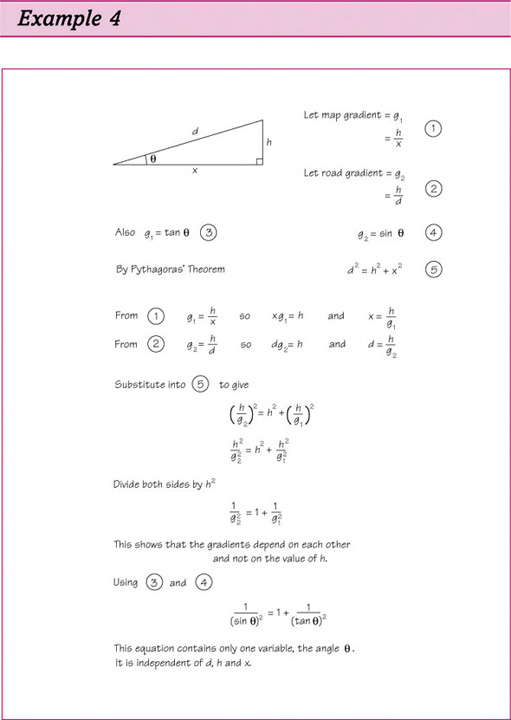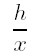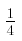Maths everywhere

This free course is available to start right now. Review the full course description and key learning outcomes and create an account and enrol if you want a free statement of participation.

Free course

# Introducing algebra

## Example 4Trying to understand this example is like trying to understand something written in a foreign language. You need to be familiar with the many symbols and signs in the same sort of way as you need to be familiar with the basic words of a language.

In this example there are symbols and signs, many of which you may not understand at present. Here are explanations of some of the symbols used in Example 4. Don't worry if you are unable to understand all the details.

• The letters d, h and x are being used to stand for the lengths of the sides of a triangle. Each one can take any sensible value—the letters are known as variables.

• The Greek letter θ (theta) is also being used as a variable, representing the size of one of the angles of the triangle—Greek letters are often used to stand for angles.

• Subscripts are used to indicate two different but related values: for example g 1 and g 2 (say g-one g-two) represent two different gradients.

• Superscripts are used to represent powersߞin particular here d 2 means d to the power of 2 or d squared.means h divided by x, just as the fractionis the same as 1 divided by 4.

• Where two letters are written together it means their values are to be multiplied. For example xg 1 is shorthand for x times g 1.

• tan and sin (say sine) stand for the trigonometric ratios, tangent and sine. Notice sin is not shorthand for s times i times n.

• = is used to denote equality. Where it occurs at the beginning of a line it means the expression that follows is the equivalent of the one above.

• Brackets have been used, along with the superscript 2, to indicate that everything in the bracket has been squared. for example,is read as ‘h divided by g all squared’.

• Several equations have been labelled ((1), (2), and so on) so that they can be referred to later.

## Activity 9 A good read

Even though you will have little idea of what the symbols represent at the moment, it is still useful to practise reading them. Read Example 4 aloud to yourself, or impress someone else by reading it to them. Remember that what you are doing is similar to reading a passage from a foreign language. As you read, try to appreciate the patterns in the symbols and begin to appreciate the underlying story. Try to read with confidence—pretend that you speak mathematics like a native!

You may have begun like this:

Let the map gradient be g-one which equals h divided by x (Equation 1)…

About half-way down comes the more difficult line:

Substitute into Equation 5 to give.

h divided by g-two all squared equals h-squared plus h divided by g-one all squared’.

Now that you have read this formidable page of algebra you may feel a little more comfortable with the ‘look’ of symbols.

MU120_1